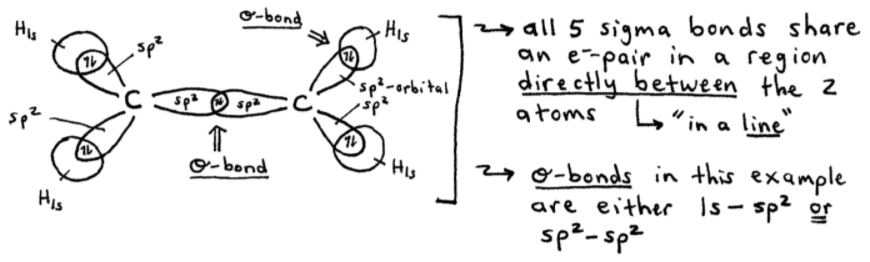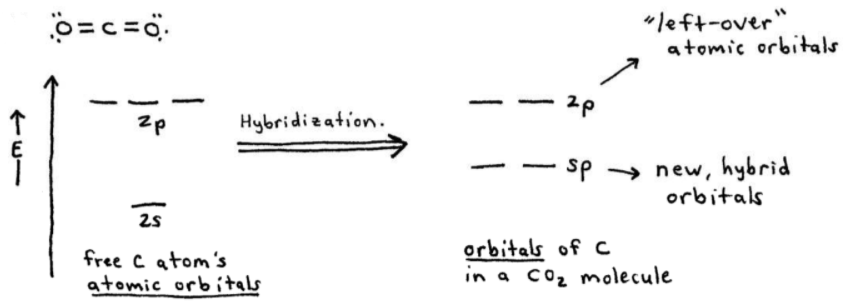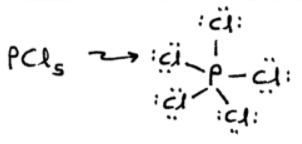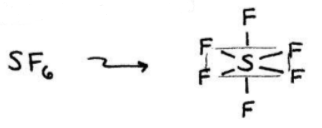# S9E2 - Types of Hybridization and Hybrid Orbitals

## sp2 hybridization

➞ Often used with double bonds or triple bonds.

ex:  C2H4 , with 12 valence electrons:In the drawing above, we see that each carbon is surrounded by 3 effective pairsAs before, an isolated carbon is 1s22s22p6 and 2s and 2p are the valence atomic orbitals.

### We need hybrid orbitals.

➞ sp3-orbitals won't work because they're tetrahedral (4 effective pairs) with 109.5° bond angles.

Here's how we can "hybridize" the one 2s and three 2p atomic orbitals into three sp2 hybrid orbitals...The 3 sp2-orbitals (hybrid orbitals) are used to share electrons in an area centered on a "line" running between the atoms.  These are called sigma bonds (σ-bonds):As you can see in the above image on the left, all 5 sigma bonds share an electron pair in a region directly between the 2 atoms.

The sigma bonds in our C2H4 example above are either 1s—sp2 or sp2—sp2.

### What about the double bond?

➞ And what about the remaining "left over" 2p atomic orbital?

➞ Well... the second bond between the two carbon atoms (the double bond) results from sharing an electron-pair using the two 2p atomic orbitals that are perpendicular to the three sp2-orbitals on each carbon.

➞ Here's a visualization of all that:These parallel p-orbitals can share an electron-pair (↑↓), which occupies the space above and below a "line" joining the carbon atoms, to form a pi bond (π-bond).

➞ So in the ethylene molecule above (C2H4), there are:
- 5 σ-bonds  (sigma bonds)
- 1 π-bond  (pi bond)

=====

## sp hybridization

➞ Used when an atom in a molecule has only 2 effective pairs around it. In the example below, the carbon (C) becomes sp-hybridized...

ex:  CO2 , with 16 valence electrons:NOTE - Both oxygens in O=C=O are sp2-hybridized because they each have 3 effective pairs around them ➞ 1 carbon, + 2 sets of lone pairs = 3 pairs.

Here's how that looks...The two 2p-orbitals on carbon are perpendicular to each other.  Additionally, each of the C=O double bonds consist of one sigma bond and one pi-bond.

=====

## dsp3 hybridization

➞ Used when the atom requires 5 effective pairs around it in a molecule.

ex:  PCl5 , with 40 valence electrons:Phosphorus' 5 dsp3-orbitals are formed from one d-orbital, one s-orbital, and three p-orbitals. They're all equivalent and are used to make 5 identical bonds to the chlorine atoms.

** a set of 5 effective pairs around an atom (i.e. Phosphorus, P) requires a trigonal bipyramidal arrangement and dsp3 hybridization.

=====

## d2sp3 hybridization

➞ Used when the atom requires 6 effective pairs around it in a molecule.

ex:  SF6 , with 48 valence electrons:Sulfur's 6 d2sp3-orbitals are formed from two d-orbitals, one s-orbital, and three p-orbitals. They're all equivalent and are used to make 6 identical bonds to the fluorine atoms.

** a set of 6 effective pairs around an atom (i.e. Sulfur, S) requires an octahedral arrangement and d2sp3 hybridization.

=====

In my next video blog post covering SECTION 9 - Covalent Bonding and Molecular Orbitals,

We'll practice doing a bunch of Hybridization Examples and Practice Problems...